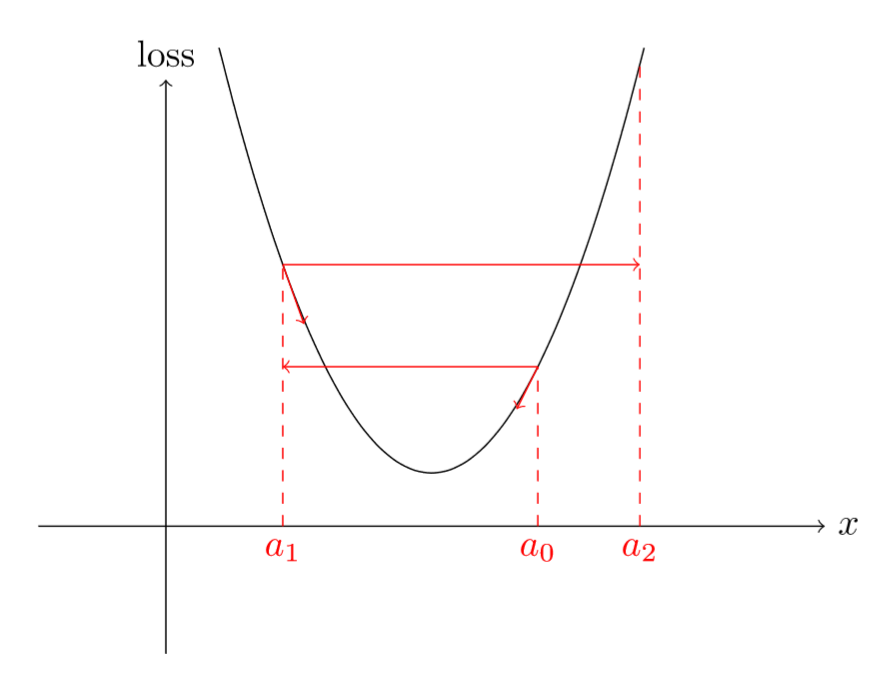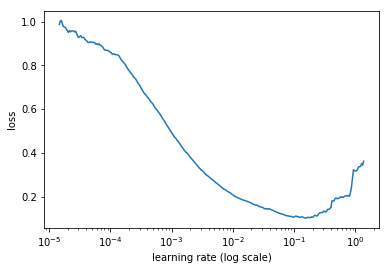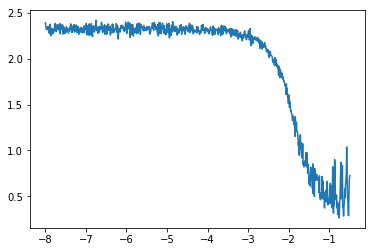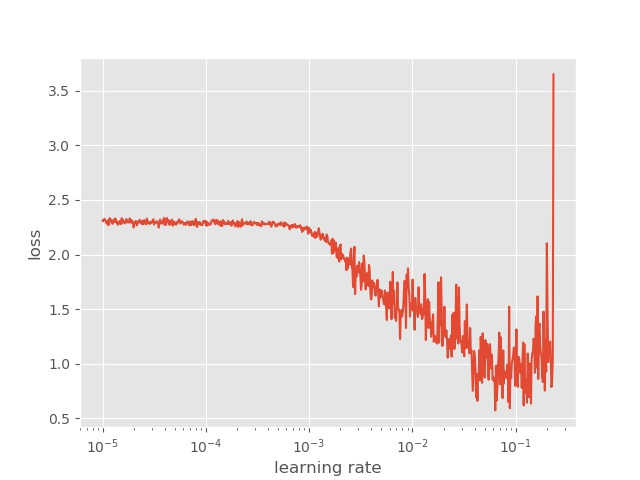## 介绍## 原理$\begin{equation*} \hbox{current loss} > 4 \times \hbox{minimum loss} \end{equation*}$

$\begin{equation*} \hbox{lr}_{i} = \hbox{lr}_{0} \times q^{i} \end{equation*}$

• $lr_0$为初始化学习率
• $q$为更新因子

$\begin{equation*} \hbox{lr}_{N-1} = \hbox{lr}_{0} \times q^{N-1} \quad \Longleftrightarrow \quad q^{N-1} = \frac{\hbox{lr}_{N-1}}{\hbox{lr}_{0}} \quad \Longleftrightarrow \quad q = \left ( \frac{\hbox{lr}_{N-1}}{\hbox{lr}_{0}} \right )^{\frac{1}{N-1}} \end{equation*}$

$\begin{equation*} \log(\hbox{lr}_{i}) = \log(\hbox{lr}_{0}) + i \log(q) = \log(\hbox{lr}_{0}) + i\frac{\log(\hbox{lr}_{N-1}) - \log(\hbox{lr}_{0})}{N-1}, \end{equation*}$

$\begin{equation*} \hbox{lr}_{i} = \hbox{lr}_{0} + i \frac{\hbox{lr}_{N-1} - \hbox{lr}_{0}}{N-1} \end{equation*}$

## 代码实践

• model: 我们需要训练的模型
• data_size: 数据集大小
• batch_size:　批大小
• fig_path: 保存图片的路径

• trainDataset：训练数据集，这里是Dataset格式
• start_lr: 开始学习率，一般设置为0.000001
• end_lr: 结束学习率，一般设置为10
• optimizer: 优化器
• epochs: 训练的epoch总数，在这里一般设置为１
• verbose:　是否打印信息，默认为1
• save: 是否保存图像